# Mathematics Questions and Answers - Form 2 Term 2 Opener Exams 2021

MATHEMATICS
FORM 2 OPENER EXAM
TERM 2  2021

INSTRUCTIONS

SECTION I:
Answer all questions in this section (40 marks)

1. Simplify without using a calculator. (3marks)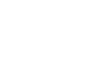2. Evaluate: (3marks)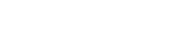3. Reduce the following expression into a single fraction. (3 marks)
4x - 5 - 2x - 1
2           6
4. Simplify: (3 marks)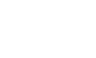5. The size of an interior angle of a regular polygon is 3x0 while exterior is (x – 20)0. Find the number of sides of the polygon. (3marks)
6. All prime numbers less than ten are arranged in ascending order to form a number.
1. Write down the number formed. (1 mark)
2. Express the number in (a) above in expanded form. (1mark)
7. Use the tables of reciprocals and square roots to evaluate.(4 marks)
0.1/0.0351 + √0.498
8. The G.C.D. and L.C.M. of three numbers are 3 and 1008 respectively. If two of the numbers are 48 and 72, find the least possible value of the third number. (3marks)
9. The ratio of Mueni’s earning to Kilonzo’s earning is 5:3. If Mueni’s earning is increased by 17% her new figure becomes Kshs. 18,000. Find the corresponding percentage change in Kilonzi’s earnings if the sum of their new earnings is Kshs. 24,600. (3 marks)
10. A straight line through the points A (2, 1) and B (4, m) is perpendicular to the line whose equation is 3y = 5-2x. Determine the value of m. (3marks)
11. Use logarithms to evaluate, correct to 4 decimal places. (4marks)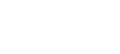12. The figure below shows a net of a solid which is not drawn to scale.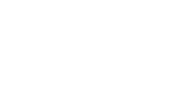Sketch the solid ABCDEF with ABCD as the base. (2 marks)
13. A number n is such that when divided by 3, 7, 11 or 13, the remainder is always one. Find the number n. (3marks)

SECTION II: (30 MARKS)
Answer any 3 questions in this section

1. A line T, which passes through the points (-3, -5) and (3, -6) and is perpendicular to a line L at the point (-2, -2).
1. Find the equation of L. (3 marks)
2. Find the equation of line T in the form ax + by = c where a, b and c are constants. (2 marks)
3. Given that another line Q is parallel to T and passes through (1, -3) find x and y intercepts of Q. (2 marks)
4. Find the points of intersection of L and Q. (3 marks)
2. Three warships A, B and C are at sea such that ship B is 500km on a bearing N30E from ship A. Ship C is 700km from ship B on a bearing of 120o.An enemy ship D is sighted 800km due south of ship B.
1. Taking a scale of 1cm to represent 100km, locate the positions of ships A, B, C and D. (4 marks)
2. Find the bearing of:
1. Ship A from D. (1 mark)
2. Ship D from C. (1 mark)
3. Use scale drawing to determine the distance between
1. D and A. (1 mark)
2. C and D. (1 mark)
3. Measure angle DAC and angle BCD (2 marks)
3. A surveyor recorded the measurements of a field in a field book as shown below. Using line AB= 260m as the baseline:
 B 170 E50 140 110 C60 F80 100 30 B50 A

1. Draw the map of the field. (4marks
2. Find the area of the field in hectares. (6marks)
4.
1. Atyang bought 3 blouses and 2 skirts for a total of Ksh.500. Awino bought 4 blouses and 5 skirts for a total of Ksh.900. Find the cost of 1 blouse and 3 skirts. (6marks)
2. A two digit number is such that the difference between the ones digit and the tens digit is 2. If the two digits are interchanged, the sum of the new and the original number is 132. Find the original number. (4 marks)

MARKING SCHEME

1.  5/ 6 x 3/6   =   5/12
1/20+ 2/3      43/60
= 5/12 x 60/43
= 25/43

2.   72/-24 - -728-24/-72
-3- -792/-72
=-3-11
=-14

3. 3(4x-5)-(2x-1)
6
12x-15-2x+1
6
10x-14
6
5x-7
3

4.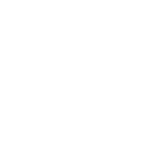5.  3xº+x-20º=180º
4xº =200º
x=50º
n=    360º     = 360º
50º-30º       20
n=18 sides

6.
1. 2357
2. 200+300+50+7

7. 0.1/0.0351 + √0.498
0.1       + √0.498
3.51 x10-1
0.2849x10+7.0569x10-1
=2.849 + 0.70569
=3.55469

8. 48=24x3
72=23x32
1008   = 7
24x32
least no= 7x3=21

9. M:K
initially mueni 5/8 x 24600=Ksh 15375
kilonzo 24600-15375=Ksh 9225
24600-18000=6600
6600-9225=-2625
Percentage change
-2625  x 100% = -28.5%
9225

10. y=-2/3x+5
m1=-2/3 m2=3/2
m-1  =
4-2     2
m-1=3
m=4

11.
 Number Log 3.45+2.626.077860.00071.741511.82146 0.783192.89542+4.845091.04168÷40.26042 3.45+2.62 =1.8215
12.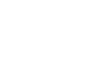13.
 3 3 7 11 13 3 1 7 11 13 7 1 1 11 13 11 1 1 1 13 13 1 1 1 1 LCM= 3x 7 x 11 x13 =3003+1
ans=3004
14.
1. mT = -5+6/-3-3=-1/6
mL=6
y+2=6(x+2)
y=6x+10

2. y+5/x+3=-1/6
6y+30 = -x-3
x+6y=-33

3. y+3/x-1=-1/6
6y+18=-x+1
x+6y=-17
x- interecpt=-17
y-intercept  -17/6

4. x+6(6x+10)=-17
37x+60-17 →x=-77/37
y=6x -77/37 + 10=-462/37 + 10
y=-92/37
(-77/37 , -92/37)
15.
1.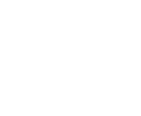2.
1. Ship A from D N35W or 3250
2. Ship D from C ,S55W or 2350
3.
1. 441km
2. 755km
4. angle DAC=61º,
angle BCD=670 ±2
16.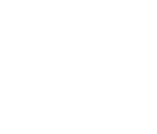1. 1/2 x 30 x 50 = 750
2. 1/2 x 80(50 + 60) = 4400
3. 1/2 x 60 x 60 = 1800
4. 1/2 x 30 x 50 = 750
5. 1/2 x 40(80 + 50) = 2600
6. 1/2 x 100 x 80 = 400
A=750+4400+1800+750+2600+400
= 14,300
= 1.43 ha
17.
1. Let the cost of a blouse be
Ksh x and a skirt be Ksh y
(3x + 2y = 500 ) x 4
(4x + 5y = 900 ) x 3
12x + 8y = 2000
12x+15y=2700
-7y=-700
-7     -7
y = 100
3x = 500 – 200
X = 100
Cost of 1 skirt & 3blouses =Ksh (100 + 3 x 100)
=Ksh.400

2. Original number = 10 x +y
New number = 10y + x
y –x = 2 .......(i)
11 x + 11y = 132
x +y = 12 .........(ii)
(i) + (ii)2y = 14
y = 7; x = 5
xy = 57

• ✔ To read offline at any time.
• ✔ To Print at your convenience
• ✔ Share Easily with Friends / Students